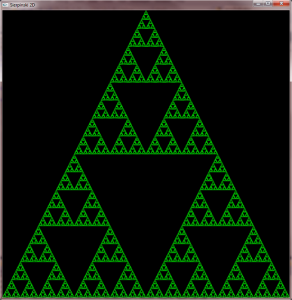# Sierpinski in OpenGLThis is just a simple 2D rendering of the famous Sierpinski gasket.

This was rendered using recursion, simple math, and GL_LINES.

``````#include "stdafx.h"
#include <stdio.h>
#include <stdlib.h>
#include <math.h>
#include "GL/glut.h"

#include<GL/glut.h>

void sierpinski2d(float vertex1[], float vertex2[], float vertex3[], int current_depth)
{
float next_vertex1,next_vertex2,next_vertex3;

//float perimeter = sqrt(vertex1);

//if(perimeter > 0.01)
//  return;

if(current_depth >= 12)
return;

glBegin(GL_LINES);
glVertex3fv(vertex1);
glVertex3fv(vertex2);
glVertex3fv(vertex3);
glEnd();

next_vertex1 = (vertex1+vertex2)/2;
next_vertex1 = (vertex1+vertex2)/2;
next_vertex1 = (vertex1+vertex2)/2;

next_vertex3 = (vertex2+vertex3)/2;
next_vertex3 = (vertex2+vertex3)/2;
next_vertex3 = (vertex2+vertex3)/2;

next_vertex2 = (vertex1+vertex3)/2;
next_vertex2 = (vertex1+vertex3)/2;
next_vertex2 = (vertex1+vertex3)/2;

sierpinski2d(next_vertex1,vertex1,next_vertex2,current_depth+1);
sierpinski2d(next_vertex1,vertex2,next_vertex3,current_depth+1);
sierpinski2d(next_vertex3,vertex3,next_vertex2,current_depth+1);
}

void myInit(void)
{

}

void display(void)
{
float vertex1={5.0,0.0,0.0};
float vertex2={-5.0,0.0,0.0};
float vertex3={0.0,5.0,0.0};
glClearColor(0.0,0.0,0.0,1.0);
glClear(GL_COLOR_BUFFER_BIT);
glColor3f(0.0,0.8,0.0);
glMatrixMode(GL_PROJECTION);
gluOrtho2D(-5.0,5.0,0.0,5.0);
glMatrixMode(GL_MODELVIEW);

sierpinski2d(vertex1,vertex2,vertex3,0);
glFlush();
}

int main(int argc,char **argv)
{
glutInit(&argc,argv);
glutInitDisplayMode(GLUT_SINGLE | GLUT_RGB | GLUT_DEPTH );
glutInitWindowSize(800,800);
glutInitWindowPosition(10,10);
glutCreateWindow("Sierpinski 2D");
myInit();
glutDisplayFunc(display);
glutMainLoop();
}
``````